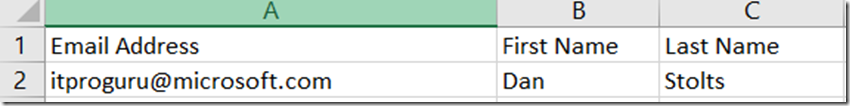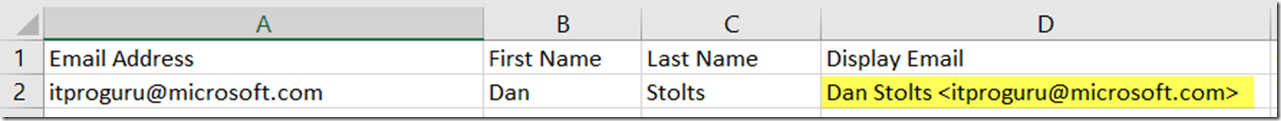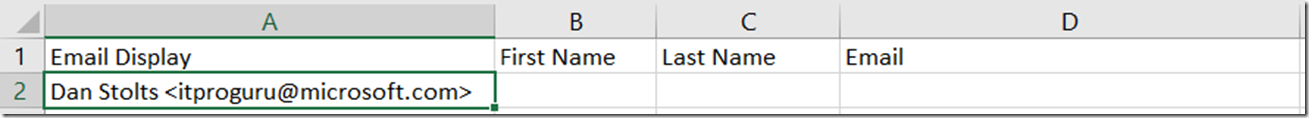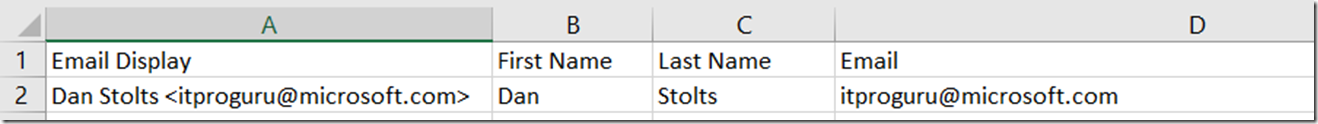# How To Cleanup Email Address in Excel, Split User Name from Email, Extract Email Address

After downloading some email addresses from a registration database, I found that people use all different sorts of formats for the emails.  Unless of course you do checking when collecting the data.  Well, I had thousands of emails that I needed to cleanup.  I turned to Microsoft Excel to help me make clean stuff up.

## How To Concatenate email address and name to create an email display name

First the the excel document looked like thisIn one scenario I want to merge that into a display name.  To do this I can use a simple formula:

=CONCATENATE(B2,” “, C2, ” “, “<“,A2,”>”)

which will give me this result:## How to extract the first and last name from an email display name

Assumption: LastName is a single word (not a multi-word name)

First Name =LEFT(A2,FIND(” “,A2,1)-1)

Last Name =MID(A2,LEN(B2)+2,FIND(” “,A2,LEN(B2))+LEN(B2)-1)

## How to Extract Email address from a text field with name and email combined

The source data looks like this:First Name =LEFT(A2,FIND(” “,A2,1)-1)

Last Name =MID(A2,LEN(B2)+2,FIND(” “,A2,LEN(B2))+LEN(B2)-1)

Email =TRIM(RIGHT(SUBSTITUTE(LEFT(A1,FIND (” “,A1&” “,FIND(“@”,A1))-1),” “, REPT(” “,LEN(A1))),LEN(A1)))

Note: The email will grab just the email address out of any text including “Please send an email to itproguru@microsoft.com and ask if we can meet on Monday morning” because it is looking for the @ sign and grabbing data between spaces. however, in our example we also have a <> as a delimiter so I want to get rid of the deliminter as well. It sure seams like there should be an easier way but in the meantime, here is what I came up with to solve the problem.

You can strip out the <> from the email with: SUBSTITUTE(A1,”>”,””),”<“,””)) however, in our formula to extract the email, and get rid of the delimiter, I have to wrap the substitute around the cell I am working with. In this case, I am not working with just a cell, but a calculation so the resulting formula looks like this:

CleanEmail =SUBSTITUTE(SUBSTITUTE(TRIM(RIGHT(SUBSTITUTE(LEFT(A11,FIND(” “,A11&” “,FIND(“@”,A11))-1),” “,REPT(” “,LEN(A11))),LEN(A11))),”>”,””),”<“,””)